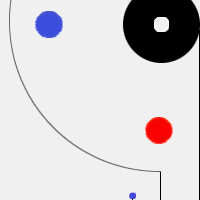Home » Audio » Group Build » 300B SET Project - Part 2: The output stage some more
300B SET Project - Part 2: The output stage some more Sun, 01 May 2005 09:20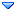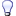Damir Messages: 1005Registered: May 2009 Illuminati (2nd Degree)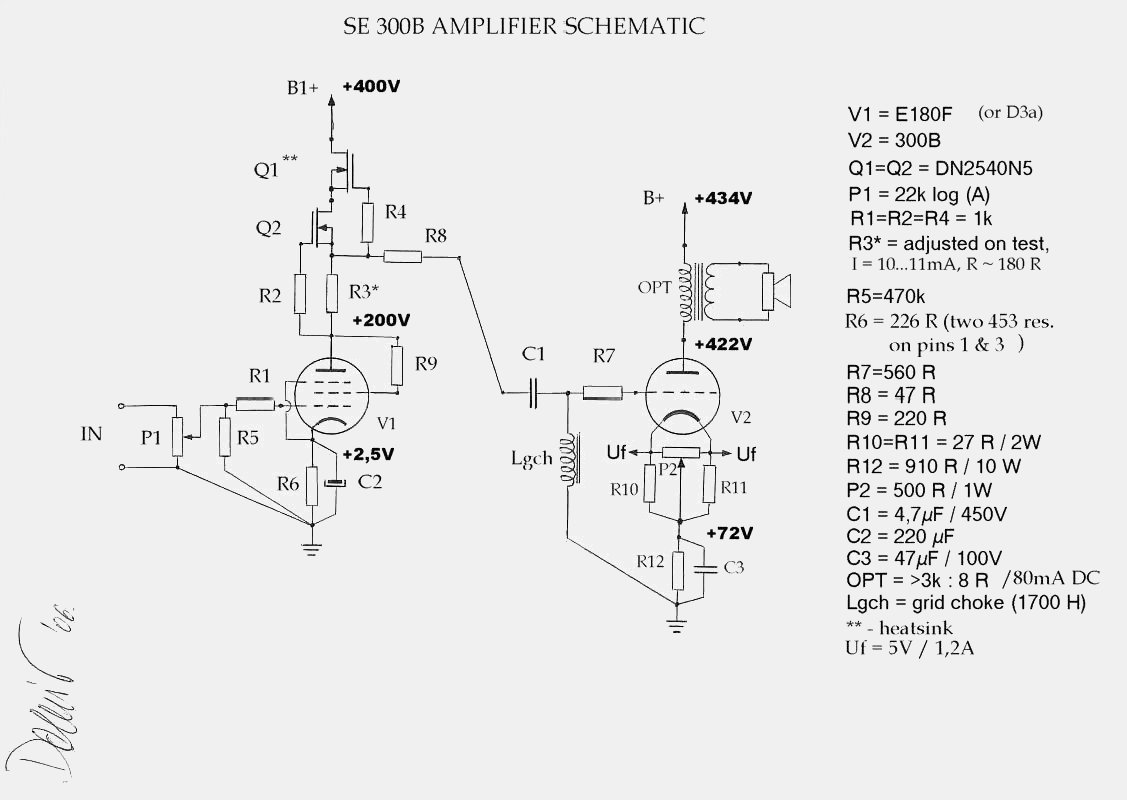At the end of the Part 1, there are some typing errors, the proper formulas are:
K2~[Ua0-(Umax+Umin)/2]/(Umax-Umin)
Pa=[(Imax-Imin)*(Umax-Umin)]/8<p>10.) We can examine various other load-lines through our OP, for example, Ra2=6kOhms and Ra3=1,5kOhms in addition with Ra1=3kOhms load line we determined (we "rounded" ra on the first "standard" value). See Fig. 5.

Using "general" formula Ra=(Ua max-Ua min)/(Ia max-Ia min), we can draw the various load-lines by the little geometry, see Fig. 6.

We have the triangle, with Ua, Ia, Ra sides. We must find a "slope" of Ra, or angle ß. Very easy - for Ra=3000 Ohms, we can draw the line through, say A`=100mA and B`=300V, using Ua min=0 and Ia min =0, Ra=300V/0,1A=3000 Ohms. Our Ra1=3k line through op. point O=350V/-70V/80mA we can draw like a parallel line to the line A`B` we just got, "preserving" the angle ß.

Back to Fig.5 - our point A1 is intersection of the Ug=0 line and Ra1=3k line, and correspond to 115V/158mA. Point B1 is intersection of the Ra1 line and Ug=-140V line, and correspond to 540V/16mA.

Verification: Ra1=(540-115)/(0,158-0,016)=2993 Ohms
Then Ua1=425Vpp=212,5Vp=150,26Vrms, and then
Pa1=Ua1^2/Ra1=150,26^2/3000=7,53W, or
Pa1=Ia1^2*Ra1=0,0502^2*3000=7,56W, or a1=Ua1*Ia1=150,26*0,0502=7,54 W, or "direct", in pp values:
Pa=[(540-115)*(0,158-0,1016)]/8 = 7,54W

In the similar way we can can determine Ra2=6k load-line, first find the temporary A2` and B2` points, say from Ra2=600V/100mA=6kOhms, parallel line through O, and then our point A2 "says" 100V/122mA/Ug=0V, and point B2 580V/42mA/-140V.

Verification: Ra2=(580-100)/(0,122-0,042)=6000 Ohms
Pa2=Ua2^2/Ra2=169,7^2/6000=4,8W, or Pa2=Ia2^2*Ra2=0,028^2*6000=4,8W.
Or Pa2=Ia2*Ra2=169,7*0,028=4,8W, or Pa2=[(580-100)*(0,122-0,042)]/8 = 4,8 W. Much lower power, but the distortion is much smaller, too:
K2~[350-(580+100)/2]/(580-100)= 2 %.

Interesting is the Ra3=1,5kOhms case, smaller then "optimum" Ra1=3k. We can see that sinusoidal input signal around -70V, from -30V up to -110V (80Vpp) "produces" resonably "clean" output Ua3, from about 230V to about 440V (210Vpp), or Pa3=Ua3^2/Ra3=74,25^2/1500=3,68 W, but then our tube "runs out of current", or in another words, we "crossed" 160 mA "upper" limit. The consequences are that with full input "swing" from Ugk=0v to Ugk=-140V, our output sinusoide is limited, or part of it is "clipped off" - large distortion (of course, we talk about "theoretical" class A1 here). Actually, Ra=1k5 condition can be reached if Rsp=4 Ohms, instead of the "nominal" 8 Ohms. See Fig. 7.

11.)We can now examine some properties of the real OPT, I have a pair of "Lundahl" LL1664/80mA, 3k:8 Ohms. It`s data are somewhat limited, but here are some:

-max. output power Pout=10W/30Hz
-primary inductance Lp=22H
-primary leakage inductance Lw=8mH
-primary "static" resistance Rw pr = 148 Ohms
-secondary "static" resistance Rw s = 0,5 Ohms
-turns ratio n=19,2:1
-We can calculate the LF power bandwith: f pb = Ra / 2*Pi*Lp = 21,3 Hz/-3dB, or in other words, OPT can "handle" half the power (5W) on the 21,7 Hz - where the load Ra is equal to the reactive impedance of the OPT. Or from 30Hz/10W (full power data), we can find -3dB power bandwith f pb = 30/1,4142021,2 Hz.
Small signal frequency response is larger, f ss ~ rp/2Pi*Lp~4,7 Hz.
he high frequency response depends on the Lw and Cw, but we don`t have the value of the winding capacitance, Cw...

-Theoretical damping factor is the ratio between the primary load and tube internal anode resistance, DF = Ra/rp, and in our case DF = 3000/650 = 4,6. But, winding resistances are actually in series with rp, and referred to the primary, Rw=Rwpr + Rw s*n^2=148+0,5*19,2^2 = 332,3 Ohms. Then our DF=3000/(650+332,3) ~ 3 times.

-Winding resistances have another bad feature - we have loss of our output power. he OPT efficiency is the ratio between the power at the speaker, Psp and "input" power Pa=Psp+Prw. we can examine both Rw s and Rw pr in a series with the speaker, and then we have Rsec = Rsp + Rw. In our case, when we Rw "reffered" to the secondary side, we have Rw=Rw s + Rw pr/n^2 = 0,9 Ohms.
E = Psp/Psec = Usp*Isec / Usec*Isec = Usp/Usec
Usp=Usec/(1+Rw/Rsp), and then E = 1/(1+Rw/Rsp).
In our case, E = 1/(1+0,9/8) = 0,9 or 90 %. It means that 10% of our theoretical Pa=7,54W (determined in chapter 10) would be heat in the winding resistances, Prw=0,75 W, and 90 % or about 6,8W would reach the speaker.

-We can try to find another OP for our 3k OPTs... For example, OP:Uak=320V, Ugk=-64,5V, Ia=80 mA. Plotting the Ra=3k line through this OP gives Ua=510-110=400Vpp, and Ia=0,15-0,02=0,13App. Then our Pa=6,5W, and K2~2,5 %, not bad...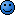Interestingly, our theoretical Ra formula gives
Ra=64,5*3,9/0,08 - 650 ~ 2,5 kOhms.

12.) CONCLUSION:
Although our analyse is simplified, we can see zhat the "theoretical" Ra formula or load line analysis where Ia is "allowed" to swing from 0-2*Ia0 gives Ra with no "current limiting" and Pa close to the max. power for chosen OP. With linear tubes in the "middle" of their Ua/Ia/Ugk characteristics, "real" graphical analysis gives the results close to the theoretical values, based on voltage source model in seies with rp, and max. current swing.
However, it is "wise" to look at the resultant Ra like the minimum, or in another words, we can use a larger Ra with somewhat lower power, but with lower distortion and larger damping factor.
In our case, we get Ra~2k8, and round it on the first "standard" value, Ra=3k like the minimum we`d like to use.Although we only "touched" some of the OPT properties, we can see that the quality of the OPT (high Lp, low Cw,Lw,Rw...) is important.

13.) F A Q :
Q) Frankly - from all those graphs, math and formulas I understand absolutely nothing. However, I`d like to find the "best" OPT primary impedance for the 300B OP I really like: Uak=350V, Ia=60mA, Ugk=-74V. Please, answer in one sentence, and one formula max!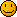A) Use my formula Ra=µ*Ugk/Ia - rp = 3,9*74/0,06 - 700 = 4110 Ohms, and "round" it on the larger "standard" value of 5 kOhms.Q) Huh, but I`d like to use OPTs I have, 2k5/60mA/17H. My buddy says that I can do it, you can`t go wrong with Ra>3*rp with 300B, and if I really need 5k OPT that I can connect 8 Ohms speaker on the 4 Ohms taps on my OPT.

A) Your buddy can be right, SE is a very subjective thing, but see "chapter" 10 once more... And yes, your 2k5 OPT is now Ra=5k OPT by changing the turns ratio with connection of the 8 Ohms speaker to the 4 hms taps. But, it`s not quite the same like the "proper" 5k OPT, for example Lp is now too small for Ra=5k, and LF can be limited and more distorted. But, you can try it, your friend can be right again, SE is very subjective thing, etc.Re: 300B SET Project - Part 2: The output stage some more [message #31077 is a reply to message #31076] Sun, 01 May 2005 16:52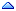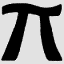Wayne Parham Messages: 17538Registered: January 2001 Illuminati (33rd Degree)

Looks like the primary will need to be 4k-5k ohms, yes? And B+ should be 450V, is that right? Thanks for all the groundwork!

Re: 300B SET Project - Part 2: The output stage some more [message #31078 is a reply to message #31076] Sun, 01 May 2005 20:14Forty2wo Messages: 163Registered: May 2009 Master
Wow. Great reading. Thank you for taking the time and effort to present it...John

Re: 300B SET Project - Part 2: The output stage some more [message #31081 is a reply to message #31077] Sun, 01 May 2005 23:41Damir Messages: 1005Registered: May 2009 Illuminati (2nd Degree)
For cathode biased amp, Uak=350V, Ugk=70V and voltage drop on the primary windind resistance Urw p = 148*0,08 = 11,84V. Then our B+ is 350+70+12=432V DC. I`d "play" with similar OPs, but this is "working" version.
And for the "optimum" Ra... depends of what OPT you have /can find:-)
I have a LL1664 (3k:8 Ohms), but better solution would be LL1623 (3k/5k6:4,8,16 Ohms, 90mA), then you have more options to try.:-)

Re: 300B SET Project - Part 2: The output stage some more [message #77209 is a reply to message #31076] Fri, 19 July 2013 03:09hungnv Messages: 1Registered: July 2013 Esquire
Damir

Can you post the picture of Part 2 again , it was disappeared.
Thanks
Re: 300B SET Project - Part 2: The output stage some more [message #80999 is a reply to message #77209] Tue, 06 January 2015 09:14horny Messages: 11Registered: May 2009 Chancellor
hello i am new here and really interested in de 300b project
but i can't see the pictures in all the 300b set projects
is this some thing in my pc or are they really gone?
Re: 300B SET Project - Part 2: The output stage some more [message #81014 is a reply to message #80999] Sun, 11 January 2015 12:09FL152 Messages: 29Registered: April 2011 Chancellor
Gone, but the final schematic is in the "Projects" files.
 Previous Topic: Build a Synthesizer Next Topic: Build From Scratch Stereo Speakers
Goto Forum:

Current Time: Sun Sep 22 01:40:27 CDT 2019